CS143 / Project 4 / Face DetectionLinear SVM, interestingly, Zoidberg is the face with highest confidence.Linear SVM, Brown computer science founders.

The goal of this project was to experiment with a face detection pipeline using support vector machines (SVMs). Like most objected detection pipelines, the face detector runs a sliding window over the test image at different scales and determines, for each scale, whether or not the window contains a face (binary classification). The basic pipeline consisted of the following steps.

1. For each example face and non face, extract features from each image.
2. Train a support vector machine to differentiate between the two classes.
3. For each test image, for each position at each scale in the image, create a window and run the classifier to determine whether or not there is a face at that location.
4. Step (3) will result in many overlapping bounding boxes for the same face, which must then be combined or suppressed into one final bounding box (non maximum suppression).

To improve the SVM accuracy, the SVM can be trained multiple times using any false detections as negative examples (mining for hard negatives) - this can potentially reduce the false postive rate, increasing accuracy.

The training data set used consisted of 6k cropped 36 x 36 faces from the Caltech Web Faces Project. Non face images used were mined from Wu et al. and the Sun scene database. The detector was benchmarked against the CMU + MIT test set, which consists of 130 images with 511 faces.

Feature Representation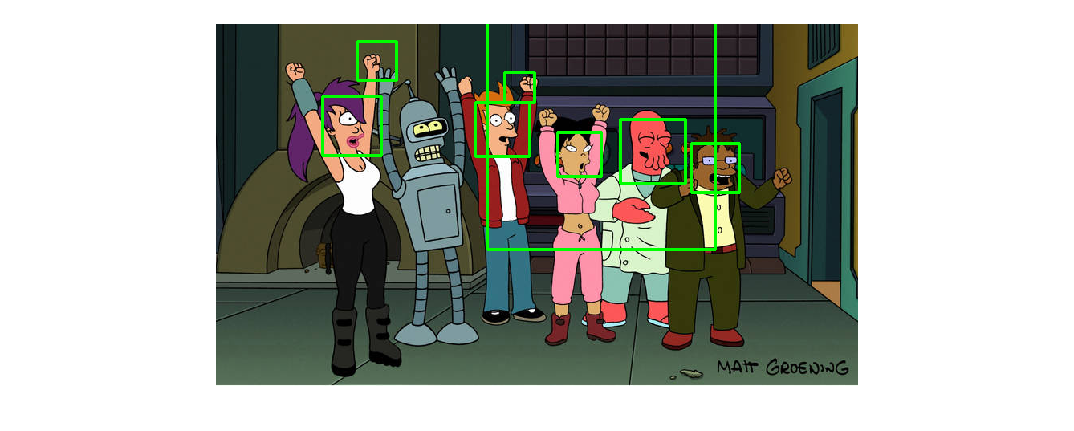Linear SVM. Suprisingly good, considering how un-face like some of the faces are.

The feature representation used for image patches makes a significant difference in detection rate. The default example (simply the image crops themselves) performed terribly. Switching the internal representation to SIFT yielded accuracies of about 37%, using the default parameters of the base implementation (1000 positive examples, linear SVM, detector step size of 4 and scale factor of 1.5, without hard negative mining)

HoG features were used as the internal representation instead of SIFT, which appeared to be relatively computationally expensive and gave relatively mediocre results (for how long it took to compute). Instead of using a prexisting HoG implementation, I decided to write my own (since there don't seem to be that many good MATLAB HoG implementations out there).

My HoG implementation gives an average accuracy of 51% (see below left), using the same settings as above - a big improvement over SIFT (which was about 37%), and better than the other HoG implementations I tried. It is also relatively fast, running the whole project pipeline from start to finish in 2 minutes (parallelization speeds this up to around 50 seconds). Using an RBF SVM, the accuracy jumps by about 10% (below right), although it is a little slower.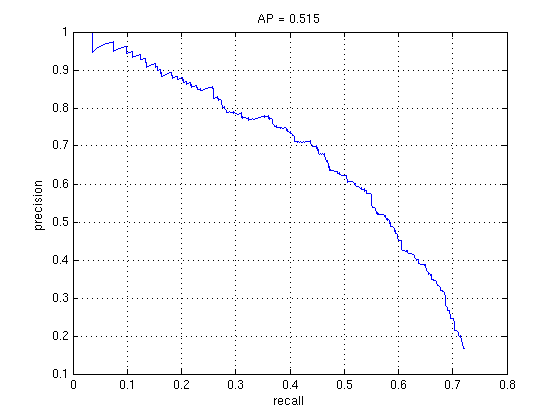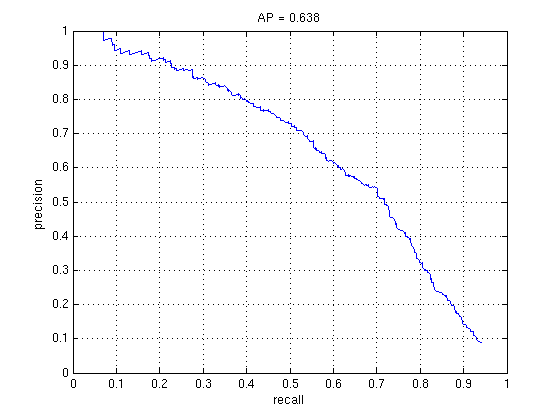1000 negative and positive training data without mining for hard negatives, Linear SVM vs RBF SVM, default detector values.

HoG Implementation

For speed, my HoG is implemented in C++ and compiled as a mex file. It follows the R-HoG descriptor as outlined in Dalal-Triggs 2005, except that the image patch is variance normalized before binning into the histogram - this makes the descriptor less sensitive to illumination changes, which gave a rough accuracy boost of around 5%.

``````
/* hog computation */
void hog(const float *imageDataIn, float *descriptorOut, const int iWidth, const int iHeight,
const int ntheta, const int ncellsx, const int ncellsy, const int ngridx, const int ngridy,
const bool signedgradient, const int interplevel)
{
...
}
``````

The basic pipeline for the HoG descriptor is:

1. Variance normalize the image patch
2. Convolve the image patch with k = [-1 0 1] both horizontally and vertically
3. Subdivide the image patch into cells
4. For each cell compute the unsigned or signed gradient angle (unsigned seems to perform better) for all the pixels in the cell as well as some neighboring cells (determined by the block size).
5. Bin the gradient magnitude into a bin based on the computed angle, weighted by a gaussian kernel centered on the cell's center (thus pixels farther away get less weight).
6. Normalize that cell's histogram

As a random and interesting (at least I think it's kind of neat) side note, the variance and mean for normalizing the image patch can be estimated in a single pass:

``````
/* image variance normalization */
void normalize(const float *imageDataIn, float *imageDataOut, const int iWidth, const int iHeight)
{
float mu = 0.f, m2 = 0.f;

// one pass variance and mean computation (Knuth 1998.  The Art of Computer Programming.)
for(int i=0;i<iWidth*iHeight;i++)
{
float delta = imageDataIn[i] - mu;
mu += delta / (float)(i+1);
m2 += delta * (imageDataIn[i] - mu);
}

const float stdev = 1.f / (sqrtf(m2 / (float)(iWidth*iHeight)) + 0.01f);

for(int i=0;i<iWidth*iHeight;i++)
{
imageDataOut[i] = (imageDataIn[i] - mu) * stdev;
}
}
``````

The HoG returns a feature which has dimension = #theta_bins x #cells_x x #cells_y

I found that the feature had best performance when the number of angular bins was best from ~ 9..12 bins, and cell size of about 4x4 pixels, similar to what the HoG authors found. However, I did not implement interpolation for histogram binning, since the block overlap coupled with gaussian weighting seems to indirectly do this already.

Hard Negative Mining

During the first training stage positive crops are used from the training data coupled with negative training crops randomly chosen from images without a face. To refine the SVM, the detector is run again on the non face scenes, and any detections (these are false positives) are used as new negative training examples to train another SVM, to decrease the false positive rate. This step can be repeated multiple times.

The strategy used for mining hard negatives was relatively simple: for each non face scene image, any detected faces were sorted by confidence, with the top results used as hard negative examples. Hard negative mining usually improved performance by about 5-10%, but seemed much more effective for the RBF SVM.

1k Random Negative vs. 1k Random + 1k Hard (Linear SVM)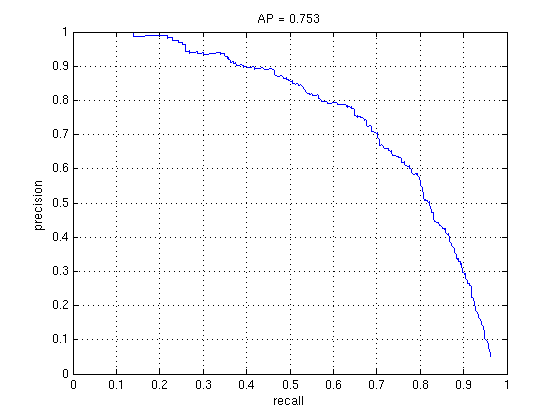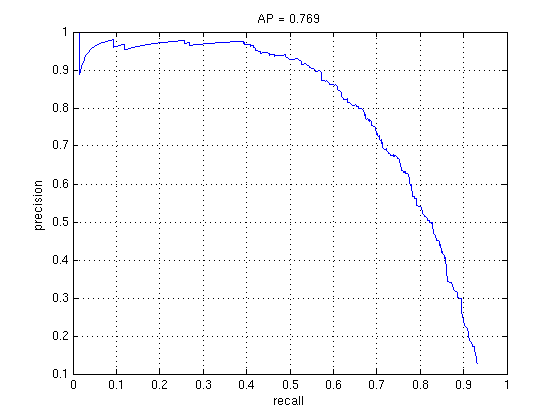1k Random Negative vs. 1k Random + 1k Hard (RBF SVM)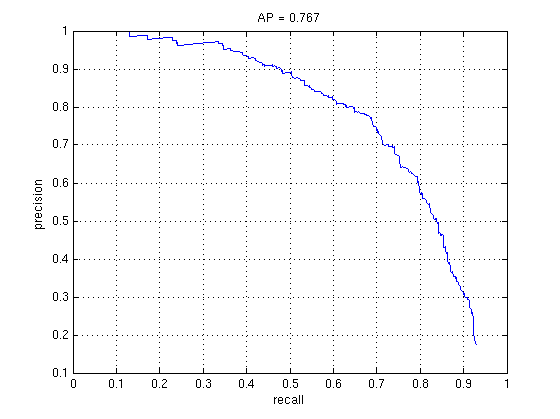Results

Below are some of the face detection results on different photos.Face detection results on the class photo.

Below are some of the more interesting face detection results on the test set. I found it surprising how well it worked on faces that weren't actual face photos, but instead drawings.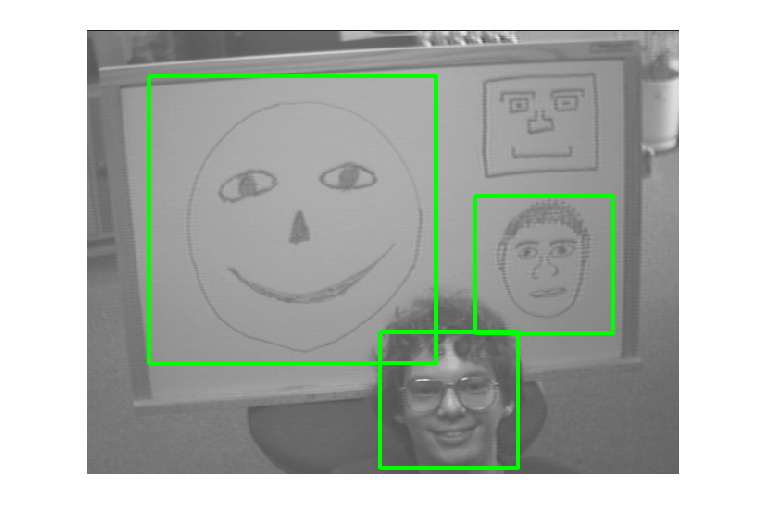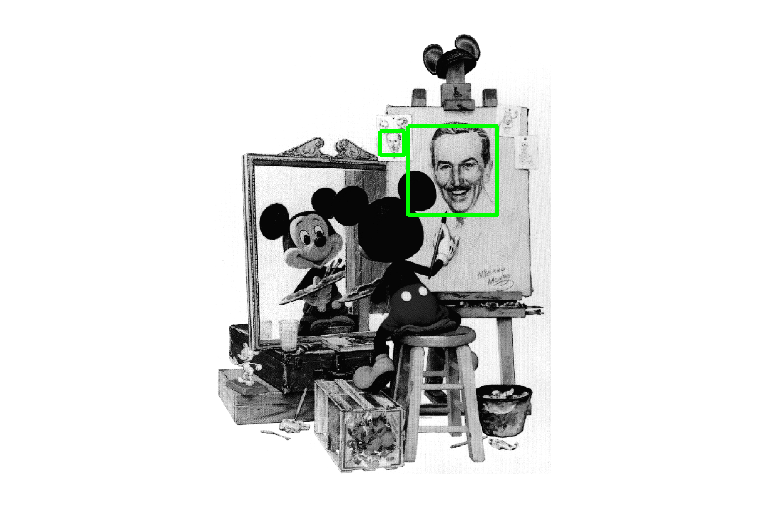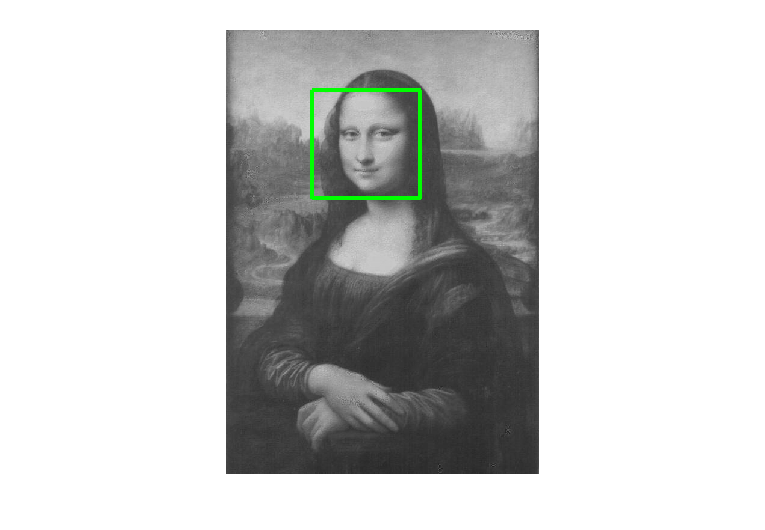Decreasing the scale factor increases the accuracy significantly, at the cost of computation time. I found that the RBF kernel was difficult to tune correctly, and couldn't get it to really beat the linear SVM by a significant amount - at best I got it to stay about even with the linear SVM (although with the RBF needing much less training data than the linear to achieve the same accuracy). Even with this advantage, however, most of my results are using the linear SVM, since it is still faster to compute, and uses much less memory.

1000 vs. 5000 Random Negatives (Linear SVM)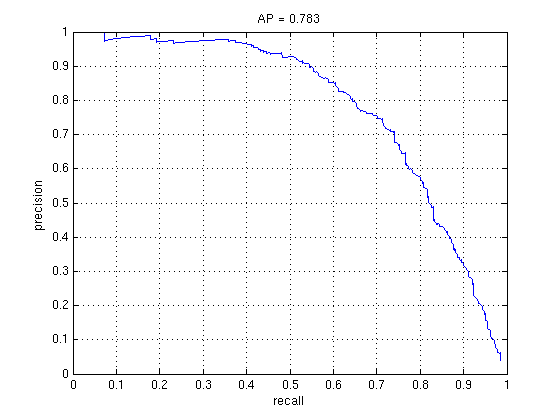2000 vs. 6000 Hard Negatives (Linear SVM)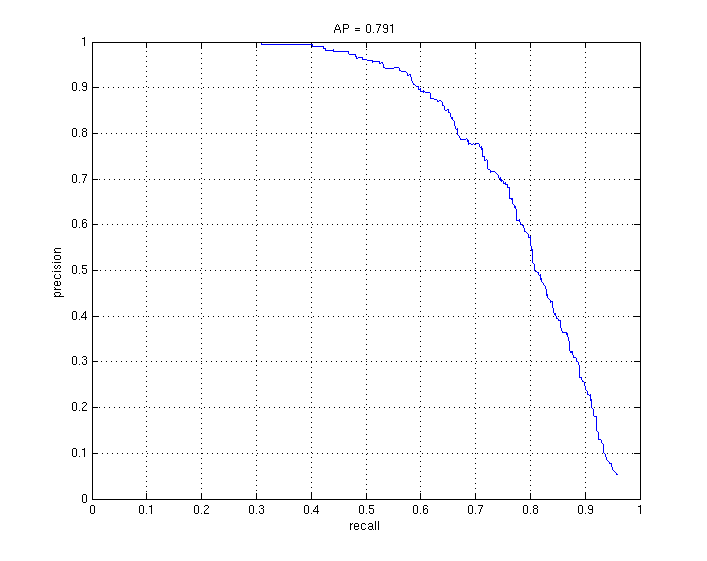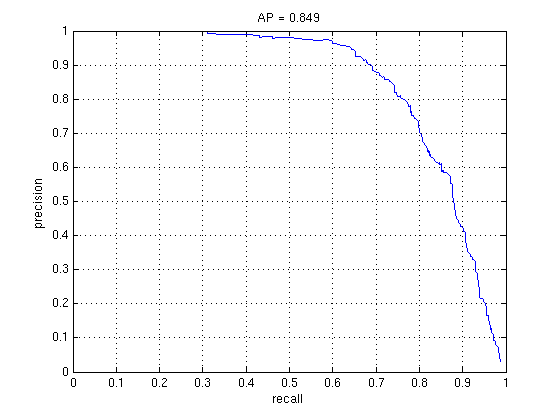Decreasing step size can also increase accuracy as well as increasing the total number of negatives.

30k Random and 30k Hard Negatives (Linear SVM) vs 1k Random and 1k Hard Negatives (RBF SVM)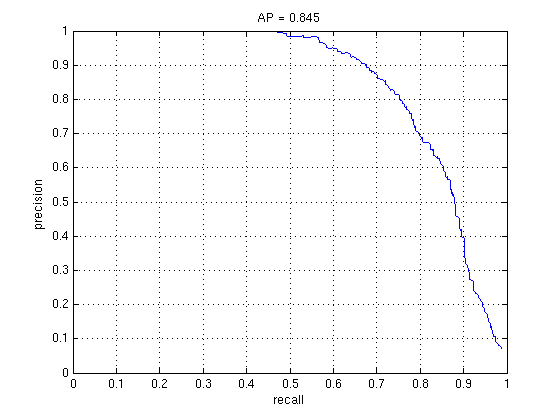It is kind of surprising how well the linear SVM managed to perform - although it should be possible to pass the linear SVM performance with the RBF kernel given careful parameter selection.